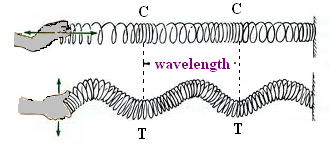Velocity of a Pulse Propagated Through a Slinky

# Materials Required:# Real Lab Procedure:

## For Transverse Waves

1. Take a slinky and place it lengthwise on the smooth surface of the table.
2. Tie one end of the slinky with the fixed hook.
3. Hold the free end of the slinky and stretch it (1 to 3 m depending upon the nature of slinky).
4. Move your hand periodically and uniformly at right angles to the length of the slinky.
5. Observe the  propagation of the wave through the slinky and observe  the formation of crests and troughs.

## For Longitudinal Waves

1. Compress the free end of the slinky periodically and observe the slinky.

Did you see an alternate compressions and rarefactions passing through the slinky?

1. Measure the wavelength by measuring the distance between two consecutive troughs (T and T) or two crests (C and C) in case of transverse wave. In case of longitudinal wave, λ is equal to distance between two consecutive compressions (C and C) or rarefactions (R and R).
2. Note the time as pulse (wave) passes through slinky for a particular distance from which we can find out the velocity of the wave.1. On jerking the free end of a slinky at a right angle to its length, there is formation of crests and troughs, along the slinky from free end toward its fixed end.
2. When regular compressions are given at the free end of the slinky, there is movement of wave from free end towards its fixed end. The movement of the wave is associated with the formation of compression and rarefactions.

## Calculations:

1.     Wavelength (λ) = the distance covered by one complete rarefaction and one complete compression.
= The distance between two consecutive crests or troughs
= ................ cm.

2.    The time taken in completing one wavelength (λ) ,T =............... second.

3.    The velocity of a pulse (wave) along slinky (v)# Simulator Procedure:

1. The simulation can be performed in two different wave types –Transverse and longitudinal.  Select your desired wave from the drop down box of ‘Select wave type’.
2. Select the desired length and pulse speed of the slinky using respective slider.
3. Now, click on the start button to do the experiment.
4. A wave or pulse is generated in the slinky
5. While moving the pulse through the slinky, a particular position of the wave is noted and the timer is started..
6. Stop the timer when the pulse reaches the specified distance.
7. Note the distance travelled and its corresponding time.
8. Calculate the velocity of pulse propagated through the slinky.

# Observations:

 Length of slinky (cm) Distance travelled by the pulse (cm ) Distance travelled by the pulse (m) Time (s) Velocity = Distance/time (m/s)

# Result:

The velocity of a pulse (wave) propagated through a stretched slinky =………………..m/s

# Precautions:

1. The slinky should have appropriate length, elasticity and flexibility.
2. One end of the slinky should be fixed properly.
3. The top of the table should be smooth.

Cite this Simulator: